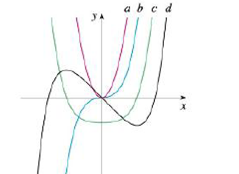Chapter 2.8, Problem 50E

Chapter
Section
Textbook Problem

The figure shows graphs of f; f', f", and f'". Identify each curve, and explain your choices.To determine

To Identify: The curves f,f,f and f on given graph and give proper explanation.

Explanation

Graph:

The given graph is shown as in Figure 1,

Observation:

Observe the graph of c and d carefully.

The point where c(x)=0 is the same point where graph of d(x) has horizontal tangent.

Recall that the derivative of a function is zero where the function has a horizontal tangent.

c(x) is the derivative of the graph d(x).

Thus, d(x)=c(x). (1)

Observe the graph of b and c carefully.

The slope of c has negative value when x<0. Only the curve b has negative value when x<0. Also, it is observed that the slope of c has positive value when x>0 while the curve b has positive value when x>0.

Thus, c(x)=b(x)

Still sussing out bartleby?

Check out a sample textbook solution.

See a sample solution

The Solution to Your Study Problems

Bartleby provides explanations to thousands of textbook problems written by our experts, many with advanced degrees!

Get Started

In Exercises 15-18, find the limit (if it exists). limx0x2x+2

Calculus: An Applied Approach (MindTap Course List)

Evaluate the integral. 56. dxx+xx

Calculus: Early Transcendentals

= _____. 0 does not exist

Study Guide for Stewart's Single Variable Calculus: Early Transcendentals, 8th

True or False: Du f(a, b) = ∇(a, b) · u.

Study Guide for Stewart's Multivariable Calculus, 8th